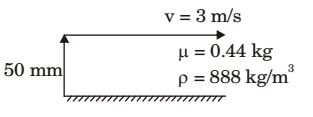## Fluid Mechanics and Hydraulic Machinery Miscellaneous

#### Fluid Mechanics and Hydraulic Machinery

1. Consider a fully developed steady laminar flow of an in compressible fluid with viscosity μ. through a circular pipe of radius R, Given that the velocity at a radial location of R/2 from the centerline of the pipe is U1, the shear stress at the wall is KμU1 /R, where K is _______.

1. NA

##### Correct Option: D

NA

1. Consider fluid flow between two infinite horizontal plates when are parallel (the gap between them being 50 mm), The top plate is sliding parallel to the stationary bottom plate at a speed of 3 m/s.
The flow between the plates is solely due to the motion of the top plate. The force per unit area (magnitude) required to maintain the bottom plate stationary is ________ N/m2.
Viscosity of the fluid μ = 0.44 kg/ms and density p = 888 kg/m3.

1.du = 7 8.0 = 60 dy 0.05

 Shear strees = τ = μ du dy

= 0.44 × 60 = 26.4 N/m²

##### Correct Option: Adu = 7 8.0 = 60 dy 0.05

 Shear strees = τ = μ du dy

= 0.44 × 60 = 26.4 N/m²

1. Oil (kinematic viscosity, υ oil = 1.0 × 10-6 m2/s) flows through a pipe of 0.5 m diameter with a velocity of 10 m/s. Water (kinematic viscosity, vw = 0.89 × 10-6 m2/s) is flowing through a model pipe of diameter 20 mm. For satisfying the dynamic similarity, the velocity of water (in m/s) is _______.

1. oil water
v = 1.0 × 10–5 m²/s v = 0.89 × 10– 6 m²/s
d = 0.5 m
d = 0.02 m
v = 10 m/sec
v =?
[Re](oil) = [Re](w)

 10 × 0.5 = V × 0.02 1.0 × 10-5 8.9 × 10-6

⇒ v = 22.25 m/s

##### Correct Option: C

oil water
v = 1.0 × 10–5 m²/s v = 0.89 × 10– 6 m²/s
d = 0.5 m
d = 0.02 m
v = 10 m/sec
v =?
[Re](oil) = [Re](w)

 10 × 0.5 = V × 0.02 1.0 × 10-5 8.9 × 10-6

⇒ v = 22.25 m/s

1. For a fully developed laminar flow of water (dynamic viscosity 0.001 Pa-s) through a pipe radius 5 cm, the axial pressure gradient is –10 Pa/m. The magnitude of axial velocity (in m/s) at a radial location of 0.2 cm is ________.

1.  u = -1 δP (R² - r²) 4μδx

 = -1 × (-10)((0.05)² - (0.002)²) 4μδx

u = 6.24 m/s

##### Correct Option: A

 u = -1 δP (R² - r²) 4μδx

 = -1 × (-10)((0.05)² - (0.002)²) 4μδx

u = 6.24 m/s

1. Air (ρ = 1.2 kg/m3 and kinematic viscosity, υ = 2 × 10-5 m2/s) with a velocity of 2 m/s flows over the top surface of a flat plate of length 2.5 m. If the average value of friction coefficient is
 Cf = 1.328 √Rex

the total drag force (in N) per unit width of the plate is _________.

1.  Cf = 1.320 √Rex

 Rex = ρvd = vd. μ υ

v = 2m/s
1 = 2.5m
υ = 2 × 10–5 m²/s
F = 1/2 CfρAυ²
A = 2.5 × 1
On substituting we get
F = 0.0159N

##### Correct Option: D

 Cf = 1.320 √Rex

 Rex = ρvd = vd. μ υ

v = 2m/s
1 = 2.5m
υ = 2 × 10–5 m²/s
F = 1/2 CfρAυ²
A = 2.5 × 1
On substituting we get
F = 0.0159N